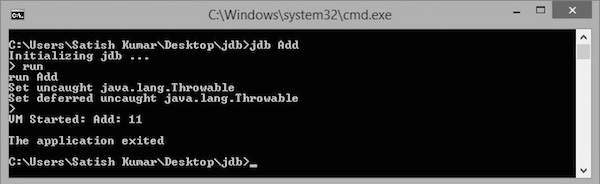JDB - Basic Commands

This chapter takes you through the basic commands of JDB. After launching a session, these commands are used for debugging a program.

The following is the list of commands used for debugging.

Name Description
help or ? The most important JDB command; it displays a list of recognized commands with a brief description.
run After starting JDB and setting the necessary breakpoints, you can use this command to start execution and debug an application.
cont Continues execution of the debugged application after a breakpoint, exception, or step.
print Displays Java objects and primitive values.
dump For primitive values, this command is identical to print. For objects, it prints the current value of each field defined in the object. Static and instance fields are included.
threads Lists the threads that are currently running.
where Dumps the stack of the current thread.

Example

Let us assume we have a sample class called Add for the following examples:

{
public int addition( int x, int y)
{
int z = x + y;
return z;
}

public static void main( String ar[ ] )
{
int a = 5, b = 6;

int c = ob.addition(a,b);
System.out.println("Add: " + c);
}
}

Compile this class Add.java using the following command: